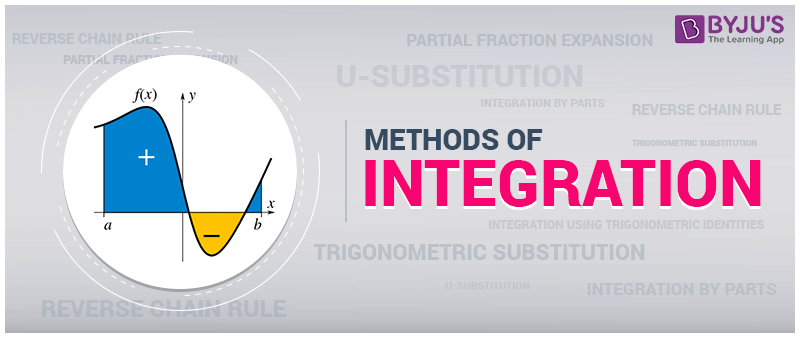# Methods of Integration

Integration is a method of adding values on a large scale, where we cannot perform general addition operation. But there are multiple methods of integration, which are used in Mathematics to integrate the functions. There are different integration methods that are used to find an integral of some function, which is easier to evaluate the original integral. Let us discuss the different methods of integration such as integration by parts, integration by substitution, integration by partial fractions in detail.## Integration Methods

The different methods of integration include:

1. Integration by Substitution
2. Integration by Parts
3. Integration Using Trigonometric Identities
4. Integration of Some particular function
5. Integration by Partial Fraction

## Integration By Substitution

Sometimes, it is really difficult to find the integration of a function, thus we can find the integration by introducing a new independent variable. This method is called Integration By Substitution.

The given form of integral function (say ∫f(x)) can be transformed into another by changing the independent variable x to t,

Substituting x = g(t) in the function ∫f(x), we get;

dx/dt = g'(t)

or dx = g'(t).dt

Thus, I = ∫f(x).dx = f(g(t)).g'(t).dt

## Integration By Parts

Integration by parts requires a special technique for integration of a function, where the integrand function is the multiple of two or more function.

Let us consider an integrand function to be f(x).g(x).

Mathematically, integration by parts can be represented as;

∫f(x).g(x).dx = f(x).∫g(x).dx–∫(f′(x).∫g(x).dx).dx

Which says:

Integral of the product of two function = (First function × Integral of second function) – Integral of [(differentiation of first function) × Integral of second function]

For deciding the first and the second functions, one can follow the ILATE rule for integration.

## Integration Using Trigonometric Identities

In the integration of a function, if the integrand involves any kind of trigonometric function, then we use trigonometric identities to simplify the function that can be easily integrated.

Few of the trigonometric identities are as follows:

$$\begin{array}{l}\sin^{2}x = \frac{1- \cos 2x}{2}\end{array}$$

$$\begin{array}{l}\cos^{2}x = \frac{1 + \cos 2x}{2}\end{array}$$

$$\begin{array}{l}\sin^{3}x = \frac{3 \sin x – \sin 3x}{4}\end{array}$$

$$\begin{array}{l}\cos^{3}x = \frac{3 \cos x + \cos 3x}{4}\end{array}$$

All these identities simplify integrand, that can be easily found out.

### Integration of Some particular function

Integration of some particular function involves some important formulae of integration that can be applied to make other integration into the standard form of the integrand. The integration of these standard integrands can be easily found using a direct form of integration method.

Also, read: Integration of some particular function

## Integration by partial fraction

We know that a Rational Number can be expressed in the form of p/q, where p and q are integers and q≠0. Similarly, a rational function is defined as the ratio of two polynomials which can be expressed in the form of partial fractions: P(x)/Q(x), where Q(x)≠0.

There are in general two forms of partial fraction:

1. Proper partial fraction: When the degree of the numerator is less than the degree of the denominator, then the partial fraction is known as a proper partial fraction.
2. Improper partial fraction: When the degree of the numerator is greater than the degree of denominator then the partial fraction is known as an improper partial fraction. Thus, the fraction can be simplified into simpler partial fractions, that can be easily integrated.